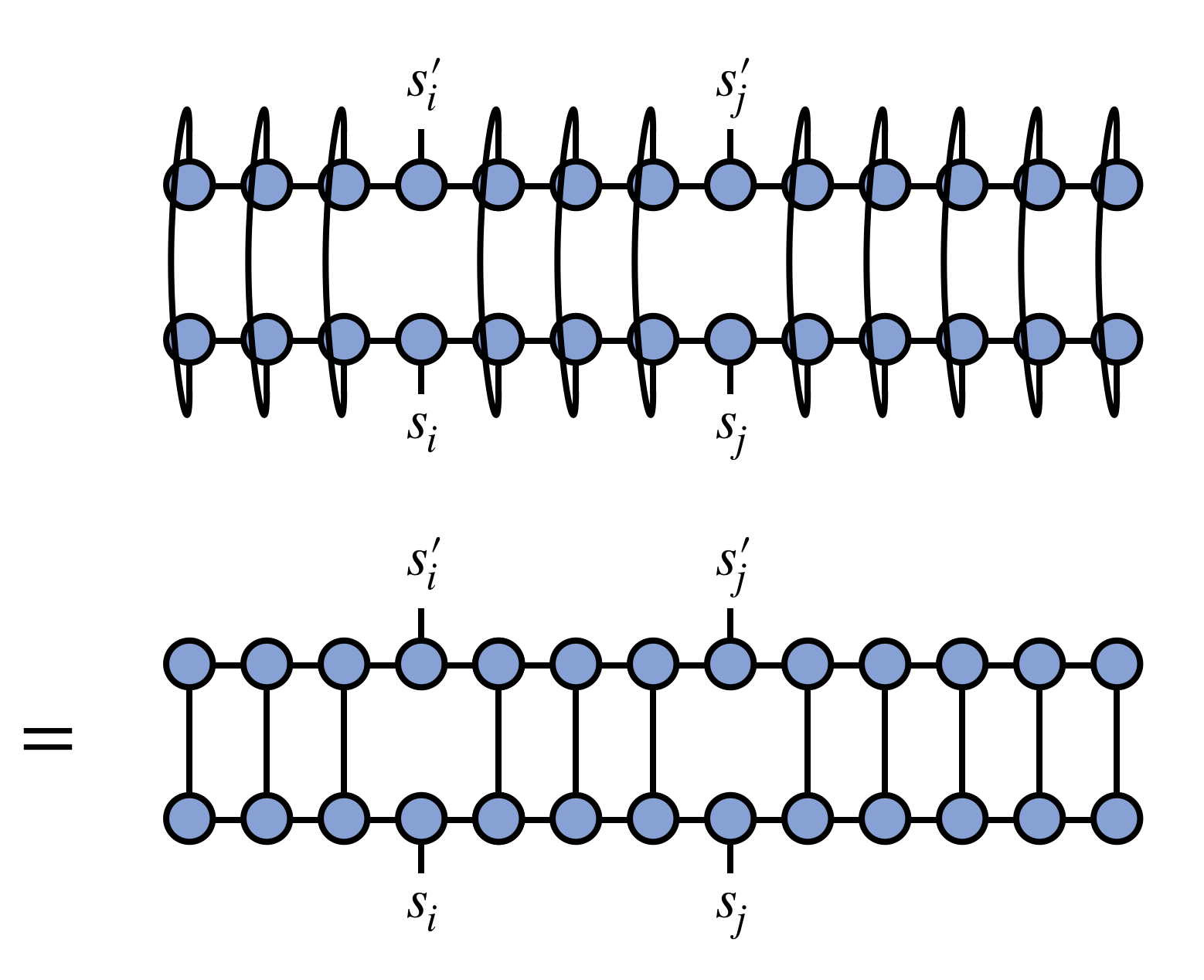## Learn to Use ITensor

main / formulas / mps_two_rdm C++v3 | C++v2 | Julia

# Compute a two-site reduced density matrix from an MPS

Say we have an MPS representing a quantum wavefunction $\Psi$ , and wish to compute the reduced density matrix

$$\rho_{s_i s_j}^{s'_i s'_j} = \text{Tr}_{\{s \neq s_i,s_j\}}[|\Psi\rangle\langle\Psi|]$$

In diagrammatic form, what we want to compute isThen we can use the following sample code to compute this quantity for fixed i and j.

### Sample code:

//Given an MPS called "psi",
//and assuming j > i
int N = 20;
auto sites = SpinHalf(N);
auto state = InitState(sites,"Up");
auto psi = randomMPS(state);

auto i = 4;
auto j = 10;

//'gauge' the MPS to site i
//any 'position' between i and j, inclusive, would work here
psi.position(i);

auto psidag = dag(psi);

auto rho = prime(psi(i),li_1)*prime(psidag(i),"Site");
for(int k = i+1; k < j; ++k)
{
rho *= psi(k);
rho *= psidag(k);
}Back to FormulasBack to Main